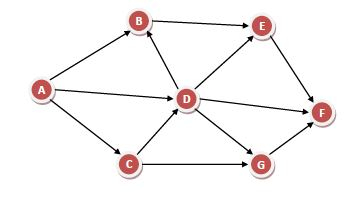# Directed Acyclic Graph (DAG)

## Definition

In computer science and mathematics, a directed acyclic graph (DAG) refers to a directed graph which has no directed cycles.

## Explanation

In graph theory, a graph refers to a set of vertices which are connected by lines called edges. In a directed graph or a digraph, each edge is associated with a direction from a start vertex to an end vertex. If we traverse along the direction of the edges and we find that no closed loops are formed along any path, we say that there are no directed cycles. The graph formed is a directed acyclic graph.

A DAG is always topologically ordered, i.e. for each edge in the graph, the start vertex of the edge occurs earlier in the sequence than the ending vertex of the edge.

## ExampleIn the above directed graph, if we find the paths from any node, say u, we will never find a path that come back to u. Hence, this is a DAG.

## Application Areas

Some of the main application areas of DAG are −

• Routing in computer networks

• Job scheduling

• Data processing

• Genealogy

• Citation graphs